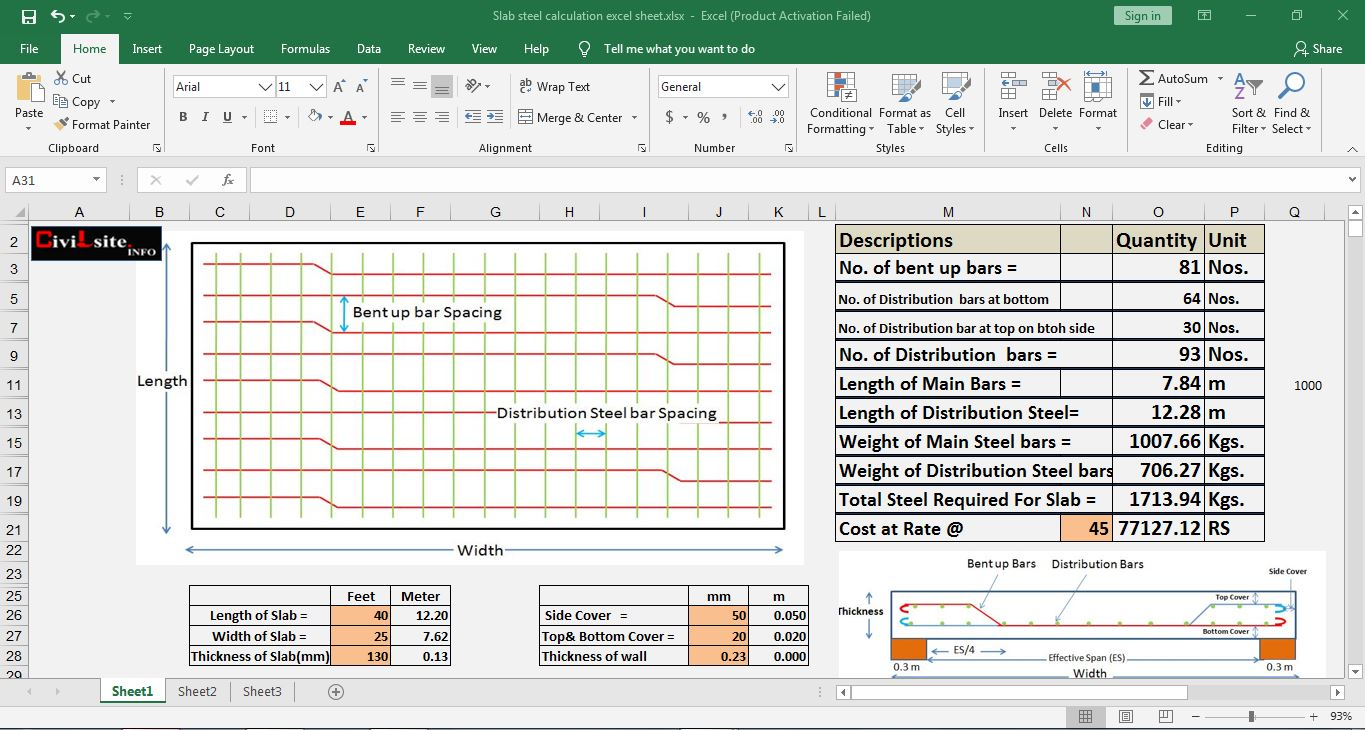# How to Calculate The Quantity of slab Reinforcement | Excel Sheet Free DownloadHow to Calculate The Quantity of slab Reinforcement

Calculating the quantity of reinforcement required for a slab involves determining the amount of both the main bars (bottom and top) and distribution bars (stirrups or links) needed to provide the required strength and stability to the structure. Here's a general step-by-step guide to calculate the quantity of slab reinforcement:

1. Understand the Design Requirements:

Before you start calculating the quantity of reinforcement, you need to have the design specifications for the slab. This includes information about the slab dimensions, the type of load it will bear, and the required strength (usually specified as a characteristic strength of concrete and a design moment or shear force).

2. Calculate Design Moments and Shear Forces:

Use the design specifications to calculate the design moments and shear forces that the slab will experience. These moments and shear forces depend on factors like the span of the slab, the type of load it will carry, and the support conditions.

3. Determine Rebar Size and Spacing:

Based on the design moments and shear forces, you can determine the required amount of reinforcement. You need to select suitable rebar sizes and spacing. The spacing of bars in the slab is governed by the structural code or design guidelines applicable in your region.

4. Calculate Total Area of Reinforcement:

Calculate the total area of reinforcement required for both the bottom (tension) and top (compression) layers. The bottom layer helps resist bending and tension, while the top layer is often used to control cracking due to shrinkage and temperature changes.

5. Calculate the Quantity of Rebars:

Multiply the total area of reinforcement by the number of bars of each size used in the slab. For example, if you're using 12mm diameter bars and the required total area is 0.1 square meters, you will need 0.1/0.0145 (approximately 6.9) bars of 12mm diameter. Since you can't have a fraction of a bar, you would round up to 7 bars.

For slabs, it's also important to provide lateral support to the main bars to prevent buckling and control shear. Calculate the length of stirrups or links required and determine the spacing based on design guidelines.

Multiply the length of stirrups or links by the number of stirrups required per unit length of the slab to get the total length needed. Then, calculate the number of stirrups or links based on the lengths and spacing.

8. Account for Lapping and Bending Lengths:

Remember to account for overlapping lengths at the joints of bars and for the length of bars that need to be bent at the ends. Lapping lengths and bending requirements are specified by design codes.

9. Consider Bar wastage:

Include a percentage for bar wastage due to cutting, bending, and fixing. The percentage can vary, but it's often around 2-5% of the total calculated length.

10. Summarize Quantities:

Summarize the quantities of all rebar sizes, lengths, and numbers required for the slab reinforcement.

11. Detailing and Drawing:
Create detailed drawings indicating the placement, arrangement, and bending of reinforcement bars.